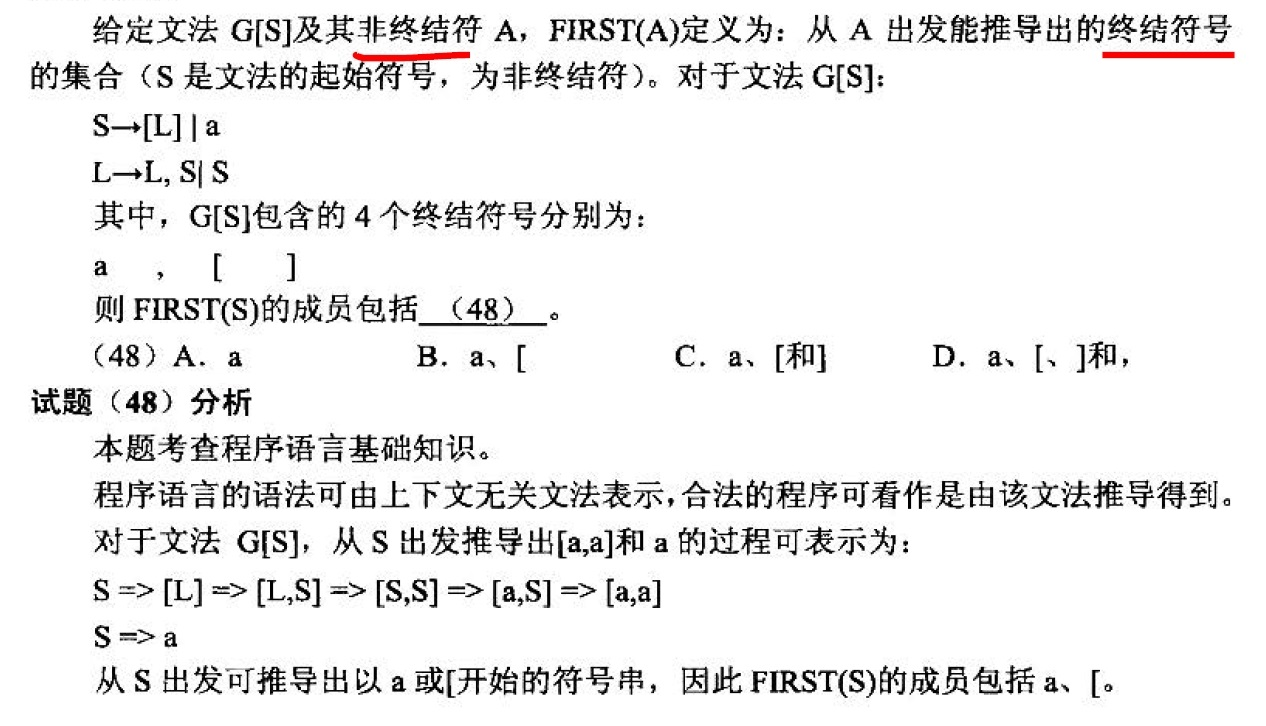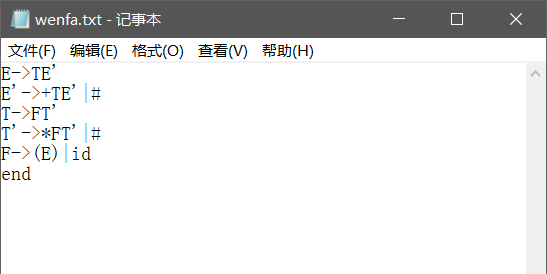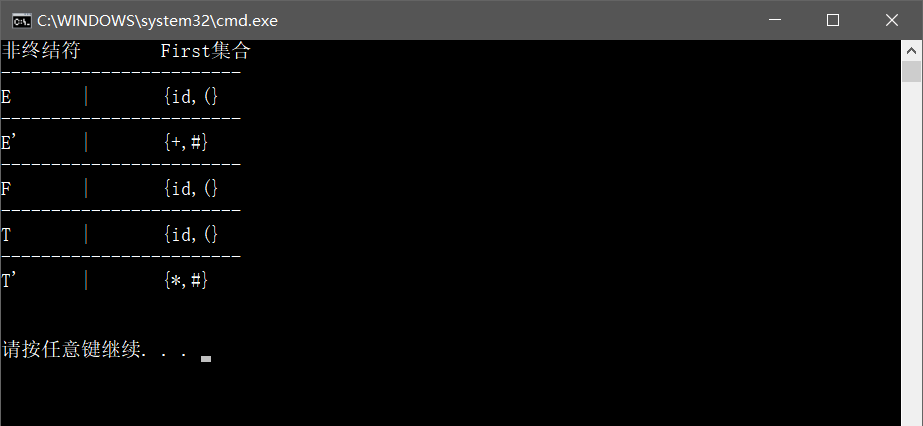• 编译原理》文法 - 终结符和非终结符 终结符 和 非终结符编译原理的文法部分是出现得最多的概念，但由于在熟悉编译原理 的人看来，这是个不值一提的简单概念，所以一般书上都没有对这两个概念做明确定义，这 ...
《编译原理》文法 - 终结符和非终结符
终结符 和 非终结符 在编译原理的文法部分是出现得最多的概念，但由于在熟悉编译原理的人看来，这是个不值一提的简单概念，所以一般书上都没有对这两个概念做明确定义，这导致很多学员在没有直正的理解其含义的情况下，开始了文法的学习，所以学起来非常吃力。
（这句话说得真好。。。就是那些无视后来人的汇编高手给偶们这些初学的带来多大的困难）
下面我们一起学习这两个概念：

终结符： 通俗的说就是不能单独出现在推导式左边的符号，也就是说终结符不能再进行 推导。

详细一点说：终结符是一个形式语言的基本符号。就是说，它们能在一个形式语法的推导规则的输入或输出字符串存在，而且它们不能被分解成更小的单位。确切地说，一个语法的规则不能改变终结符。例如说，下面的语法有两个规则： x -> xa x -> ax 在这种语法之中，a是一个终结符，因为没有规则可以把a变成别的符号。不过，有两个规则可以把x变成别的符号，所以x是非终结符。一个形式语法所推导的形式语言必须完全由终结符构成。

非终结符： 不是终结符的都是非终结符。非终结符可理解为一个可拆分元素，而终结符是不可拆 分的最小元素。

非终结符是可以被取代的符号。一个形式文法中必须有一个起始符号；这个起始符号属于非终结符的集合。

判断注意： （1）只要存在有 S→L ，则 S 必然是个非终结符 （2）逗号，[，]，（，） 这5个都是终结符 （3）一般书上把非终结符用大写字母 表示，而终结符用小写字母表示。
识别符号： 就是开始符。由文法产生语言句子的基本思想是： 从识别符号开始，把当前产生的符号串中的非终结符号替换为相应规则右部的符号串，直到 最终全由终结符号组成。这种替换过程称为推导或产生句子的过程，每一步成为直接推导或 直接产生。
例如：
有文法G2[S]为： S->Ap S->Bq A->a A->cA B->b B->dB 则表示：S 为开始符，S，A，B 为非终结符，而p,q,a,b,c,d 为终结符
还想继续进阶吗？
来道题？顶得住吗？展开全文• 一、非终结符： 1、非终结符可以再分成更细的东西。 2、不是终结符的都是非终结符。非终结符可理解为一个可拆分元素，而终结符是不可拆分的最小元素。终结符号就是语言中用到的基本元素，名词、动词、形容词、助词...
一、非终结符：
1、非终结符可以再分成更细的东西。
2、不是终结符的都是非终结符。非终结符可理解为一个可拆分元素，而终结符是不可拆分的最小元素。终结符号就是语言中用到的基本元素，名词、动词、形容词、助词等等基本语言单位。
二、终结符：
1、终结符直接就代表一个意思，比如关键字if就不能再分成i和f了。
2、通俗的说就是不能单独出现在推导式左边的符号，也就是说终结符不能再进行推导。非终结符则是"语法"中用到的元素，除非谈论"语法"，一般交谈语言中并不会用到非终结符。比如：主语、短语、词组、句子。
展开全文• 题目目的：熟练掌握自上而下的语法分析方法，并能用程序实现。 要求： 例如. 使用的文法如下： E->TE' ...2， 终结符为 小写字母和符号（+、*）(#代表空串) 3， 推导符号为-> 4， 用end结束文法。 5，
博客已经迁移到 https://leerw.github.io，欢迎来访，共同交流进步
题目
目的：熟练掌握自上而下的语法分析方法，并能用程序实现。
要求：
例如. 使用的文法如下：
E->TE'
E'->+TE'|#
T->FT'
T'->*FT'|#
F->(E)|id
编写First函数，实现其求解过程。
提示：
1，  非终结符为大写字母；或后面带'的大写字母
2，  终结符为 小写字母和符号（+、*）(#代表空串)
3，  推导符号为->
4，  用end结束文法。
5，  不针对特定文法，编写求first函数。

思路
从文件中读取文法，对每一行将其拆分成两个部分：箭头左边的非终结符和箭头右边的对应产生式；再将产生式按其中的'|'分隔符拆分成若干个对应单词；对每个单词进行考察，若首字母为非终结符则递归寻找First集合，若为终结符，则加入到此非终结符对应的First集合中去。

代码
/*
*Programed by Lee_rw_cs on 2017/05/04
*Find the first set of a set of grammar
*eg.
*E->TE'
E'->+TE'|#
T->FT'
T'->*FT'|#
F->(E)|id
*/

#include <iostream>
#include <algorithm>
#include <fstream>
#include <map>
#include <set>
#include <string>

using namespace std;

//全局变量

set<string> non_terminal;                                                       //存放非终结符
set<string> productions;                                                        //存放产生式
std::map<string, string> match_map;                                             //存放非终结符和其对应的产生式的文法的键值对
std::map<string, set<string>> first;                                            //string:非终结符;set<string>:非终结符所对应的first集合

void divide_words(string grammar, map<string, string>& match_map) {
for (int i = 0; i < (int)grammar.length(); ++i) {
if (grammar[i] == '-' && grammar[i + 1] == '>') {
/* code */
string left = grammar.substr(0, i);                                 //一句文法的左边即非终结符
string right = grammar.substr(i + 2, grammar.length() - 1);         //一句文法的右边即非终结符对应的产生式
non_terminal.insert(left);                                          //插入非终结符集合里
productions.insert(right);                                          //插入产生式集合里
match_map.insert(make_pair(left, right));                           //将一句文法里的非终结符和其对应的文法作为键值对插入到匹配map里
break;
}
}
}
/*将被'|'隔开的产生式拆分成对应多个的单词*/
void divide_right(string grammar_right, set<string>& small_right) {
/*或许可以用grammar.find_first_of一个一个找|，然后用substr分开子串，最后再insert到small_right中去*/
size_t found = grammar_right.find('|');
if (found != string::npos) {
int i = 0;
string temp = "\0";
while ((size_t)i < grammar_right.length()) {
if (grammar_right[i] != '|') {
temp += grammar_right[i];
i = i + 1;
}
else {
i = i + 1;
small_right.insert(temp);
temp = "\0";
}
if (i == grammar_right.length()) {
small_right.insert(temp);
temp = "\0";
}
}
}
else {
small_right.insert(grammar_right);
}
}
/*对每个非终结符non_term寻找它的非终结符集合first*/
void find_first(string non_term, set<string>& first) {
set<string> or_words;                                               //存放产生式中被'|'隔开的单词
auto search = match_map.find(non_term);
if (search != match_map.end()) {
divide_right(search->second, or_words);
//匹配非终结符是否在or_words的开头
for (set<string>::iterator i = or_words.begin(); i != or_words.end(); i++) {
for (set<string>::iterator j = non_terminal.begin(); j != non_terminal.end(); j++) {
if ((*i).find(*j) == 0) {                               //在or_words[i]的开头找到了一个非终结符
//递归寻找非终结符j的first集合
find_first((*j), first);
}
else {                                                  //在or_words[i]的开头如果没有找到非终结符，即终结符
if ((*i) >= 'a' && (*i) <= 'z') {
first.insert(*i);
}
switch ((*i)) {
case '(':
first.insert(string("("));
break;
case ')':
first.insert(string(")"));
break;
case '+':
first.insert(string("+"));
break;
case '*':
first.insert(string("*"));
break;
case '#':
first.insert(string("#"));
break;
default:                                            //如果没有匹配到符号的话就把这个单词插入到first集合中
//first.insert(*i);
break;
}
continue;                                           //找到之后跳出循环，避免进行多余的遍历浪费时间
}
}
}
}
}

int main() {
/*读取文法文件*/
const char* filename = "wenfa.txt";
ifstream inFile(filename);
if (!inFile) {
cout << "\nFiled to open file " << filename;
return -1;
}
string st = "\0";
char buf;
while (!inFile.eof()) {
inFile.getline(buf, 100);
st = buf;
if (strlen(buf) == 0 || st == "end") {
break;
}
divide_words(st, match_map);                                        //对每一行文法进行分析找出非终结符和对应的产生式
}
inFile.close();
/*遍历非终结符集合，为每个非终结符寻找first集合*/
for (set<string>::iterator i = non_terminal.begin(); i != non_terminal.end(); ++i) {
set<string> the_first;                                              //当前非终结符的first集合
find_first(*i, the_first);
first.insert(make_pair(*i, the_first));
}
cout << "非终结符" << "\t" << "First集合" << endl;
for (map<string, set<string>>::iterator i = first.begin(); i != first.end(); i++) {
cout << "------------------------" << endl;
cout << i->first << "\t|\t";
cout << "{";
//倒序输出first集合中的元素与文法中出现的顺序保持一致
for (set<string>::reverse_iterator j = (i->second).rbegin(); j != (i->second).rend(); j++) {
cout << *j << ",";
}
cout << '\b';
cout << "}";
cout << endl;
}
cout << endl << endl;
return 0;
}
wenfa.txt运行结果：展开全文c++
• #pragma once #include #include #include #include #include #include .../*----------------------------------------全局变量存放终结符和非终结符--------------------------------------
还是先把代码给贴出来，回头再找时间写篇文章总结一下，分析一下数据结构和算法
#pragma once
#include <iostream>
#include <algorithm>
#include <fstream>
#include <map>
#include <set>
#include <string>
#include <stack>

using namespace std;

/*----------------------------------------全局变量存放终结符和非终结符---------------------------------------------------*/
set<string> non_terminal;                                                       //存放非终结符
set<string> productions;                                                        //存放产生式
std::map<string, string> match_map;                                             //存放非终结符和其对应的产生式的文法的键值对
std::map<string, set<string>> first;                                            //string:非终结符;set<string>:非终结符所对应的first集合
std::map<string, set<string>> follow;                                           //string:非终结符;set<string>:非终结符所对应的follow集合
bool is_not_changing = false;

void divide_words(string grammar, map<string, string>& match_map) {
for (int i = 0; i < (int)grammar.length(); ++i) {
if (grammar[i] == '-' && grammar[i + 1] == '>') {
/* code */
string left = grammar.substr(0, i);                                 //一句文法的左边即非终结符
string right = grammar.substr(i + 2, grammar.length() - 1);         //一句文法的右边即非终结符对应的产生式
non_terminal.insert(left);                                          //插入非终结符集合里
productions.insert(right);                                          //插入产生式集合里
match_map.insert(make_pair(left, right));                           //将一句文法里的非终结符和其对应的产生式作为键值对插入到匹配map里
break;
}
}
}
/*将被'|'隔开的产生式拆分成对应多个的单词*/
void divide_right(string grammar_right, set<string>& small_right) {
/*或许可以用grammar.find_first_of一个一个找|，然后用substr分开子串，最后再insert到small_right中去*/
size_t found = grammar_right.find('|');
if (found != string::npos) {
int i = 0;
string temp = "\0";
while ((size_t)i < grammar_right.length()) {
if (grammar_right[i] != '|') {
temp += grammar_right[i];
i = i + 1;
}
else {
i = i + 1;
small_right.insert(temp);
temp = "\0";
}
if (i == grammar_right.length()) {
small_right.insert(temp);
temp = "\0";
}
}
}
else {
small_right.insert(grammar_right);
}
}
/*对每个非终结符non_term寻找它的非终结符集合first*/
void find_first(string non_term, set<string>& first) {
set<string> or_words;                                               //存放产生式中被'|'隔开的单词
auto search = match_map.find(non_term);
if (search != match_map.end()) {
divide_right(search->second, or_words);
//匹配非终结符是否在or_words的开头
for (set<string>::iterator i = or_words.begin(); i != or_words.end(); i++) {
for (set<string>::iterator j = non_terminal.begin(); j != non_terminal.end(); j++) {
if ((*i).find(*j) == 0) {                               //在or_words[i]的开头找到了一个非终结符
//递归寻找非终结符j的first集合
find_first((*j), first);
}
else {                                                  //在or_words[i]的开头如果没有找到非终结符，即终结符
if ((*i) >= 'a' && (*i) <= 'z') {
first.insert(*i);
}
switch ((*i)) {
case '(':
first.insert(string("("));
break;
case ')':
first.insert(string(")"));
break;
case '+':
first.insert(string("+"));
break;
case '*':
first.insert(string("*"));
break;
case '#':
first.insert(string("#"));
break;
default:                                            //如果没有匹配到符号的话就把这个单词插入到first集合中
//first.insert(*i);
break;
}
continue;                                           //找到之后跳出循环，避免进行多余的遍历浪费时间
}
}
}
}
}

//对非终结符的follow集进行初始化，开始符号的follow集初始化成{$}，其余的初始化成空集 void initial_follow() { for (set<string>::iterator i = non_terminal.begin(); i != non_terminal.end(); i++) { if (i == non_terminal.begin()) { set<string> startFollow; startFollow.insert("$");
auto pair = make_pair(*i, startFollow);
follow.insert(pair);
}
set<string> temp_follow;
auto pair = make_pair(*i, temp_follow);
follow.insert(pair);
}
}
//判断一个非终结符的first集合中是不是含有空串#
bool first_contains_null(set<string> &first) {
auto find = first.find("#");
if (find != first.end()) {
return true;
}
return false;
}
//判断一个字符串str是否是非终结符，如果是返回true,否则返回false
bool is_non_terminal(string str) {
auto find = non_terminal.find(str);
if (find != non_terminal.end()) {
return true;
}
return false;
}

bool is_letter(char a) {                                                        //是否是小写字母
if (a >= 'a' && a <= 'z') {
return true;
}
return false;
}

bool is_cap_letter(char a) {
return (a >= 'A' && a <= 'Z') ? true : false;
}

//返回一个产生式的右部str的最后一个终结符或者非终结符
string find_last(string &str) {

if ("\0" == str) {
return "\0";
}
if ('\'' == str.at(str.size() - 1)) {
string s = str.substr(str.size() - 2, 2);
str = str.substr(0, str.size() - 2);
return s;
}
else if (is_letter( str.at(str.size() - 1) ) && is_letter( str.at( str.size() - 2) ) ) {
string s = str.substr(str.size() - 2, 2);
str = str.substr(0, str.size() - 2);
return s;
}
else {
string s = str.substr(str.size() - 1, 1);
str = str.substr(0, str.size() - 1);
return s;
}
}

int cal_follow_total_size() {                                                   //计算所有follow集合的总size
int total_size = 0;
for (map<string, set<string>>::iterator i = follow.begin(); i != follow.end(); i++) {
total_size += i->second.size();
}
}

void find_follow(std::map<string, set<string>>& Follow) {
while (!is_not_changing) {
int fomer_size = cal_follow_total_size();
for (std::map<string, string>::iterator i = match_map.begin(); i != match_map.end(); i++) {//对每一个产生式进行遍历
set<string> or_words;
string left = (*i).first;                                       //左边的非终结符A
string right = (*i).second;                                     //右边的产生式A->b1b2b3B...
divide_right(right, or_words);
for (set<string>::iterator j = or_words.begin(); j != or_words.end(); j++) {
set<string> temp = Follow.find(left)->second;
string str;
string word = *j;
for (; word != "\0"; ) {
str = find_last(word);
if (!is_non_terminal(str)) {                        //是终结符
temp.clear();
temp.insert(str);
}
else {
for (set<string>::iterator k = temp.begin(); k != temp.end(); k++) {
if ("#" != (*k)) {
(Follow.find(str)->second).insert(*k);
}
}
if (!first_contains_null(first.find(str)->second)) {
temp = first.find(str)->second;
}
else {
for (set<string>::iterator m = first.find(str)->second.begin(); m != first.find(str)->second.end(); m++) {
temp.insert(*m);
}
}
}
}
}
}
//判断是否发生变化
int latter_size = cal_follow_total_size();
is_not_changing = fomer_size == latter_size ? true : false;
}
}
int main() {
/*读取文法文件*/
const char* filename = "wenfa.txt";
ifstream inFile(filename);
if (!inFile) {
cout << "\nFiled to open file " << filename;
return -1;
}
string st = "\0";
char buf;
while (!inFile.eof()) {
inFile.getline(buf, 20);
st = buf;
if (strlen(buf) == 0 || st == "end") {
break;
}
divide_words(st, match_map);                                        //对每一行文法进行分析找出非终结符和对应的产生式
}
inFile.close();
/*遍历非终结符集合，为每个非终结符寻找first集合*/
for (set<string>::iterator i = non_terminal.begin(); i != non_terminal.end(); ++i) {
set<string> the_first;                                              //当前非终结符的first集合
find_first(*i, the_first);
first.insert(make_pair(*i, the_first));
}
cout << "非终结符" << "\t" << "First集合" << endl;
for (map<string, set<string>>::iterator i = first.begin(); i != first.end(); i++) {
cout << "-------------------------" << endl;
cout << i->first << "\t|\t";
cout << "{ ";
//倒序输出first集合中的元素与文法中出现的顺序保持一致
for (set<string>::reverse_iterator j = (i->second).rbegin(); j != (i->second).rend(); j++) {
cout << *j << ", ";
}
cout << "\b\b }";
cout << endl;
}
cout << endl;
initial_follow();
find_follow(follow);
cout << "非终结符" << "\t" << "Follow集合" << endl;
for (map<string, set<string>>::iterator i = follow.begin(); i != follow.end(); i++) {
cout << "------------------------------" << endl;
cout << i->first << "\t|\t";
cout << "{ ";
//倒序输出first集合中的元素与文法中出现的顺序保持一致
for (set<string>::reverse_iterator j = (i->second).rbegin(); j != (i->second).rend(); j++) {
cout << *j << ", ";
}
cout << "\b\b }";
cout << endl;
}

return 0;
}

展开全文• 由于书中没有对终结符和非终结符给出明确的定义，导致看的昏昏沉沉，因此专门搜索资料理清楚它们的含义。 1.终结符 终结符是一个形式语言的基本符号。就是说，它们能在一个形式语法的推导规则的输入或输出字符串...语言
• 编译原理》文法 - 终结符和非终结符 终结符 和 非终结符编译原理的文法部分是出现得最多的概念，但由于在熟悉编译原理的人看来，这是个不值一提的简单概念，所以一般书上都没有对这两个概念做明确定义，这导致...
• 文法 维基百科的解释： 在形式语言理论中，文法（为了避免歧义，常称作“形式文法”）是形式语言中字符串的一套产生式规则。这些规则描述了如何用语言的字母表生成符合语法的有效的字符串。...终结符、非终...编译器
• ## 终结符和非终结符

万次阅读 多人点赞 2015-06-14 13:14:50
终结符和非终结符编译原理的文法部分是出现得最多的概念，但由于在熟悉编译原理 的人看来，这是个不值一提的简单概念，所以一般书上都没有对这两个概念做明确定义，这 导致很多学员在没有直正的理解其含义的...
• 学习完编译原理课程后，对编译原理章节知识点的概括总结，类似于科目大纲，只不过是自己写的用来串联自己脑中的知识点。 第一章：编译程序基本概念 List item sad 二、使用步骤 1.引入库 代码如下（示例）： ...编程语言
• 编写程序：输入任意文法，统计Vn,Vt Vt表示为终结符，Vn表示非终结符 汇编原理的第一个代码实现c语言
• 终结符 和 非终结符编译原理的文法部分是出现得最多的概念，但由于在熟悉编译原理的人看来，这是个不值一提的简单概念，所以一般书上都没有对这两个概念做明确定义，这导致很多学员在没有直正的理解其含义的情况...编程语言
• 终结符：不是一个语法成分，表示他本身，是不需要被解释的符号。 非终结符：表示一个语法成分，是需要被进一步解释的符号。 EBNF范式中， 所有用尖括号 <>括起来的符号都是“非终结符”， 在 ::= 左边的是...
• 文章目录 前言 一、pandas是什么？ 二、使用步骤 1.... 2....一、终结符定义 ...这里前提是主语不可再分，你如果说主语是由字母或者数字组成，那么你也可以认为字母为终结符。 二、非终结符是什么 ...
• VN表示非终结符 VT表示终结符 P表示产生式 S表示开始符2，老师上课说的编译原理的阶段说的是：词法分析，语法分析，语义分析，中间代码生成，代码优化，目标代码生成 其中 语法分析，语义分析，中间代码生成称为 ...优化
• 编译原理总结 编译原理总结 文章目录编译原理总结前言一、编译的过程二、前端1.词法分析2.语法分析2.1 LL分析2.2 LR分析3.语义分析4. 中间代码5. 后端5.1 指令选择5.2 活跃性分析5.3 寄存器分配5.4 数据流分析5.5 ...
• 编译原理实验报告实验名称 Chomsky文法类型判断(Recognizing the type of the Chomsky grammar)实验时间 2014年11月1号院系 计算机科学与技术学院软件工程(1)班班级 软件工程(1)班学号姓名 周媛媛试验目的输入：一组...
• 编译原理学习笔记     1.文法的定义（上下文无关文法）       (1) 上下文无关文法由四个元素组成 一个终结符号集合 一个终结符号集合 一个产生式集合 指定一个...
• 1.什么是文法文法是用于描述语言的语法结构的形式规则。...在某个形式语法之中，终结符和非终结符是两个不交的集合。通俗的说,终结符号就是语言中用到的基本元素,一般不能再被分解;名词,动词,形容...
• 编译原理的应用 准备系统地整理学习编译原理的相关知识。虽然这部分知识很多时候非常抽象，却能够很高的提高程序员内在的修养。如果每一种程序语言是一套招式，那么编译原理就是内功。不管程序语言如何变，都摆脱...开发工具 编程语言
• ## 编译原理实验二

千次阅读 2019-01-06 21:15:16
编译原理实验二 1.实验内容： 要完成Decaf编译器的语法分析工作，即用bison工具生成一个语法分析程序，对词法分析输出的单词符号串（终结符串）进行自底向上的分析，并依次输出用来进行归约的语法规则。 2.实验详解 ...Yacc 自底向上
• int是终结符还是非终结符 重点 (Top highlight)How do you take your internet? I, for one, like it private and secure. 您如何上网？ 我，一方面，喜欢它的私密性和安全性。 Unfortunately, it does not matter ...
• G=（VT,VN,P,S）,其中VT是终结符集合，VN是非终结符集合（表示语法成分的符号） VT∩VN=Ø VT∪VN=文法符号集 P是产生式集合，包含了将终结符和非终结符连接成串的方法 产生式的一般形式： α→β（α定义为β） ①...
• 文章目录待解决的问题tips易错提醒二义性前缀 待解决的问题 总结消除二义性的套路 DFA ⟶\longrightarrow...first集就是非终结符星推导得到的第一个终结符或者ϵ\epsilonϵ的集合。当 α⟶∗ϵ\alpha \longrigh...
• 编译原理学习笔记, 超万字的编译原理知识总结..................